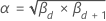# Selection of the optimal tree in CART® Classification

Depending on your selection, the optimal tree is the tree that yields the minimum misclassification cost or the smallest tree with a misclassification cost within a multiple of standard errors of the minimum misclassification cost. The determination of the optimal tree depends on the validation method.

For more details on the model validation methods and complexity parameters, see Breiman, Friedman, Olshen and Stone (1984)1.

## Model validation methods

Model summary statistics, such as the area under the ROC curve, tend to be optimistic when you calculate them with the same data that you use to fit a model. Model validation methods leave a portion of the data out of the model fitting process, then calculate statistics that evaluate the performance of the model on the omitted data. Model validation techniques provide a better estimate of how well models perform on new data. The misclassification cost from the omitted data is the criterion for the selection of the optimal tree. Minitab offers two validation methods for predictive analytics techniques: k-fold cross-validation and validation with a separate test data set.

## The optimal tree with K-fold cross-validation

K-fold cross-validation is the default method in Minitab when the data have 5000 cases or less. With this method, Minitab portions the data into K subsets. The subsets are called folds. K-fold cross validation tends to work well with data sets that are relatively small compared to data sets that work well with a test data set. Because the process repeats K times, cross-validation is usually slower than validation with a test data set.

### K-fold cross-validation procedure

To complete k-fold cross-validation, Minitab produces 1 + k sequences of subtrees. One sequence of subtrees, the master sequence, uses the entire training data set. The other k sequences are for the k folds. For each fold, the sequence of subtrees uses (k – 1)/k of the cases in the training data set.

Each sequence consists of a finite sequence of nested subtrees. Each fold has a finite sequence of complexity parameters αdααd + 1 that correspond to the largest tree and the subtrees in the sequence. The sequence that is for the full data set has complexity parameters βd ββd + 1where d = 0, 1, ... D, where β0 is the parameter for the largest tree in the sequence.

For any subtree in the master sequence, assume the corresponding complexity parameters are βd and βd + 1 . Let. Then, Minitab uses this alpha to find the k corresponding subtrees from the k folds. For each fold, calculate the misclassification cost for the subtree using the formula in Methods and formulas for the model summary in CART® Classification. The average misclassification cost across k folds is the estimated misclassification cost for the subtree in the master sequence. Repeat the calculation of the estimated misclassification cost for each subtree in the master sequence. The procedure identifies the subtree with the minimum average misclassification cost. The tree with the minimum misclassification cost or the smallest tree with a misclassification cost within a multiple of standard errors of the misclassification cost becomes the optimal tree in the results.

## The optimal tree with a separate test data set

In validation with a test data set, a portion of the data is set aside for validation. This portion of the data is the training data set. First, Minitab fits all the trees with the training data set. Then, Minitab calculates either the mean square error or the absolute deviation for the test data set for each tree. The tree with the optimal value of the criterion for the test data set is the optimal tree.

## The optimal tree with no validation

Without any validation, Minitab uses the entire data set to grow the sequence of subtrees. The subtree with the most terminal nodes has the minimum misclassification cost and is the optimal tree.

1 Breiman, Friedman, Olshen & Stone. (1984). Classification and Regression Trees. Boca Raton, Florida: Chapman & Hall/CRC.
By using this site you agree to the use of cookies for analytics and personalized content.  Read our policy